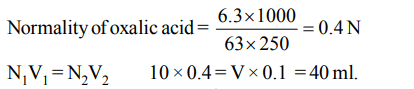## Some Basic Concepts of Chemistry Questions and Answers Part-4

1. The number of molecules in 16 g of methane is
a) $3.0 × 10^{23}$
b) $\frac{16}{6.02} × 10^{23}$
c) $6.02 × 10^{23}$
d) $\frac{16}{3.0} × 10^{23}$

Explanation: 16 g CH4 is 1 mol. Hence number of molecules = Avogadro number = 6.023 × 1023

2. 50 ml 10 N $H_{2}SO_{4}$   , 25 ml 12 N HCl and 40 ml 5 N $HNO_{3}$    were mixed together and the volume of the mixture was made 1000 ml by adding water. The normality of the resultant solution will be
a) 2 N
b) 1 N
c) 3 N
d) 4 N

Explanation:3. A molal solution is one that contains 1 mole of a solute in
a) one litre of the solvent
b) 1000 g of the solvent
c) one litre of the solution
d) 22.4 litres of the solution

Explanation: Molal solution contains 1 mole of solute in 1000 g solvent.

4. A 100 ml solution of 0.1 N HCl was titrated with 0.2 N NaOH solution. The titration was discontinued after adding 30 ml of NaOH solution. The remaining titration was completed by adding 0.25 N KOH solution. The volume of KOH required for completing the titration is
a) 16 ml
b) 32 ml
c) 35 ml
d) 70 ml

Explanation:5. An aqueous solution of 6.3 g of oxalic acid dihydrate is made up to 250 ml. The volume of 0.1 N NaOH required to completely neutralise 10 ml of this solution is
a) 20 ml
b) 40 ml
c) 10 ml
d) 4 ml

Explanation:6. The percentage of nitrogen in urea is about
a) 85
b) 46
c) 18
d) 28

Explanation:7. How much of NaOH is required to neutralise $1500cm^{3}$ of 0.1 N HCl? (Na = 23)
a) 60 g
b) 4 g
c) 6 g
d) 40 g

Explanation:8. The volume of water to be added to $100 cm^{3}$ of 0.5 N $H_{2}SO_{4}$   to get deci normal concentration is
a) $400cm^{3}$
b) $450cm^{3}$
c) $500cm^{3}$
d) $100cm^{3}$

Explanation:9. 250 ml of a sodium carbonate solution contains 2.65 grams of $Na_{2}CO_{3}$. If 10 ml of this solution is diluted to one litre, what is the concentration of the resultant solution? (mol. wt. of $Na_{2}CO_{3}=106$    )
a) 0.1 M
b) 0.01 M
c) 0.001 M
d) $10^{-4}M$10. 3.92 g of ferrous ammonium sulphate crystals are dissolved in 100 ml of water. 20 ml of this solution requires 18 ml of potassium permaganate during titration for complete oxidation. The weight of $KMnO_{4}$  present in one litre of the solution of